# Fire Safety Assessment of High-Rise Buildings Based on Fuzzy Theory and Radial Basis Function Neural Network

Fire Safety Assessment of High-Rise Buildings Based on Fuzzy Theory and Radial Basis Function Neural Network
School of Construction Engineering, Yiwu Industrial & Commercial College, Yiwu 322000, China

Corresponding Author Email:
mingbiaoxu@163.com
Page:
267-274
|
DOI:
https://doi.org/10.18280/isi.250215
10 November 2019
|
Accepted:
5 February 2020
|
Published:
15 May 2020
| Citation

OPEN ACCESS

Abstract:

Featuring complex functions, dense population, and large span, high-rise buildings are an iconic product of economic and technical growths in modern society. Fire poses an imminent threat to high-rise buildings. Once a high-rise building catches fire, the loss of life and property will be incalculable. However, the traditional assessment methods for fire safety of high-rise buildings are incapable of handling the complex influencing factors. To solve the problem, this paper combines the fuzzy logic inference system and radial basis function neural network (RBFNN) into an intelligent assessment method mimicking the nonlinear inference process of fire safety experts. Firstly, the factors affecting the fire safety of high-rise buildings were quantified, and the relevant rating standard was established. Next, the classic statistics were transformed into fuzzy indices, using the fuzzy logic system. To avoid the local minimum trap, the RBFNN was adopted to replace the traditional backpropagation neural network (BPNN), and integrated with the fuzzy logic system, creating an adaptive fuzzy-RBFNN to assess the fire safety of high-rise buildings. The proposed network was trained by numerous expert evaluation samples, and verified by examples. The simulation results show that the proposed network could mimic the nonlinear inference process of fire safety experts, and evaluate the fire safety of high-rise buildings in real time with little error. The research results provide new insights on the application of artificial intelligence (AI) in fire safety assessment.

Keywords:

high-rise buildings, fuzzy logic system, radial basis function neural network (RBFNN), fire safety

1. Introduction

China is currently the second largest economy in the world. With the rapid growth of economy and technology, more and more high-rise buildings have been constructed [1, 2]. Fire poses an incalculable threat to high-rise buildings. In less severe cases, fire may burn buildings and furniture. If things get worse, people could be injured or killed by fire [3-5]. The current fire safety standards for buildings cannot satisfy the demand of modern buildings for fire safety. It is impossible to assess the overall fire safety of high-rise buildings based on the score of a single item in the standards. The assessment of fire safety experts, who have rich experience in engineering, is more reliable and accurate. But such experts are hard to find, and take a long time to make the assessment. Besides, there is no mathematical formula that accurately describes the expert assessment of fire safety, which is a typical nonlinear reasoning process. Therefore, it is urgent to develop an artificial intelligence (AI)-based method to simulate the nonlinear reasoning by experts, and to realize fast and accurate assessment of the fire safety of high-rise buildings.

In the past few decades, many cities have constructed high-rise buildings to accommodate the fast-growing urban population. Meanwhile, many fire accidents broke out in high-rise buildings, causing serious casualties and property losses. These accidents are resulted from the lack of maintenance and poor management. The reliability of fire safety measures in buildings is greatly affected by the level of maintenance. In other words, the service time of fire safety components depends on the interaction between the maintenance strategy and its environment . However, building owners and managers always want to keep maintenance cost as low as reasonably possible. Hence, it is necessary to evaluate fire safety in advance and prioritize relevant tasks. In addition to maintenance requirements, the government should issue legal notices to building owners, requiring them to improve fire safety, and establish a reasonable rating system to evaluate and classify the safety level of buildings. Currently, the safety level of buildings could be analyzed based on existing codes and basic physical and thermodynamic models. Nonetheless, none of these thermodynamic simulation methods are sufficiently comprehensive, consistent, or convenient for fire safety assessment. After all, most high-rise buildings are multi-story complex buildings, with incomplete records of construction methods and materials.

Against this backdrop, various methods have been developed to assess the fire safety of high-rise buildings. Watts  was the first to elaborate the fire safety rating system in a comprehensive manner. Zheng et al.  introduced the backpropagation neural network (BPNN) to assess the fire safety of large shopping malls. Chow  proposed a 10-point safety rate system to evaluate fire safety, according to the fire safety requirements on high-rise buildings in Hong Kong. Han et al.  integrated data collection, risk distribution calculation, and evaluation reroute planning, and put forward a comprehensive real-time evacuation route planning method for high-rise building fire; this method could evaluate fire status and make evacuation plans. Considering the inaccuracy of the information to be processed, Watts  included fuzzy set theory [12, 13] into the fire safety assessment system. Based on multi-criteria and fuzzy logic, Paralikas  presented a rapid assessment method for chemical substances and devices. Extended from fuzzy theory, the above fire safety assessment systems [15-18] face an inevitable problem: the difficulty of pairwise comparisons increases with the number of attributes or experts.

To overcome the problem, Lo  proposed an inaccurate information processing method based on reliability interval and grey relational model; this method allows experts to assign interval scores rather than fixed scores to attributes. Later, Lo  developed a fuzzy fire safety assessment mechanism, in which fire safety attributes are identified by Delphi method, and the weight of each attribute was evaluated through analytic hierarchy process (AHP) . Drawing on the statistical theory of fuzzy set, Xia et al.  to mathematically analyzed the stochasticity in fire risk assessment of high-rise buildings, calculated the total weight of all indices, and evaluated the fire risk by the linear weighted model. Since it is difficult to identify risk factors amidst uncertainties, fire safety could not be assessed easily based on event tree or fault tree. For high-rise buildings, it is hard to apply statistical inference to fire safety assessment, because historical data are often insufficient or too indicative.

The fire safety of high-rise buildings is affected by various complex factors. This highlights the importance of fire safety experts, known for their rich experience and knowledge, in fire safety assessment of high-rise buildings. Because the previous analyses are mostly qualitative, this paper attempts to analyze the fire safety of high-rise buildings in a quantitative fashion. First, a fire safety evaluation system was established for high-rise buildings, using the advantages of the AI in system learning and automatic pattern recognition. The fire protection system, fire extinguishing system, the safety evacuation system, and other factors were quantified under the established system. On this basis, an adaptive fuzzy-radial basis function neural network (RBFNN) was proposed, and trained with numerous expert evaluation samples. The trained evaluation network was verified by examples. The results show that our network could judge whether high-rise buildings meet the fire safety requirements of modern buildings rapidly and accurately.

2. Fire Safety Assessment System for High-Rise Buildings

2.1 Evaluation index system

For high-rise buildings, the prevention of fire largely hinges on a comprehensive fire safety assessment system. From the perspective of fire prevention and control, this paper mainly considers four primary indices in the assessment of fire safety, namely, fire protection system, fire extinguishing system, safety evacuation system, and other factors.

(1) Fire protection system

Fire protection system can effectively curb the spread of fire, making it possible for personnel to evacuate from the building. This system could be further decomposed into piping shafts and building components; smoke exhaust and ventilation systems; fire power supply and power distribution.

(2) Fire extinguishing system

Fire extinguishing system can detect the fire in the early stage. Then, the system will issue an alarm to remind personnel to evacuate and escape, and activate the firefighting equipment to put out or contain the early fire. It is very important to evaluate the success probability PS of the fire extinguishing system:

$P_{S}=P_{R E} \times P_{O L A} \times P_{O P R}$      (1)

where, PRE, POLA, POPR are the response efficiency, online availability, and operability, respectively.

(3) Safety evacuation systemwhere, PRE, POLA, POPR are the response efficiency, online availability, and operability, respectively.

In high-rise buildings, the safe escape of personnel from fire directly depends on the rational design of safe evacuation passages and the effective organization of evacuees. The safety evacuation system could be broken down into: fire elevators; fire lanes or the sites for aerial platform fire trucks; safe passages; evacuation distances; evacuation stairs; evacuation signs. Among them, evacuation signs are essential in high-rise buildings: the signs guide personnel to escape safety from the fire scene. The intact rate A0 of the safety evacuation system reflects the level of fire hazard:

$A_{0}=\frac{n_{1}}{n_{1}+n_{2}}=\frac{n_{1}}{n} \times 100 \%$     (2)

where, n1, n2 and n are the number of intact basic units, the number of incomplete basic units, and the total number of basic units.

(4) Other factors

If the fire is serious, a fire brigade is needed to put out the fire. The sooner the fire brigade reaches the scene, the more likely it is to extinguish the fire. The other factors include firefighting skills; personnel management; maintenance situation; surrounding environment.

2.2 Quantification of fire safety assessment indices for high-rise buildings

For simplicity, the fire safety assessment system for high-rise buildings can be expressed as a set:

$u=\left\{u_{1}, u_{2}, u_{3}, u_{4}\right\}$      (3)

where, u is the composite evaluation index; u1_u4 are the four primary indices, namely, fire protection system, fire extinguishing system, safety evacuation system, and other factors, respectively.

The qualitative secondary indices under each primary index can be expressed as:

$u_{1}=\left\{u_{11}, u_{12}, u_{13}, u_{14}\right\}$     (4)

$u_{2}=\left\{u_{21}, u_{22}, u_{23}, u_{24}, u_{25}, u_{26}\right\}$     (5)

$u_{3}=\left\{u_{31}, u_{32}, u_{33}, u_{34}, u_{35}, u_{36}\right\}$    (6)

$u_{4}=\left\{u_{41}, u_{42}, u_{43}, u_{44}\right\}$     (7)

Table 1. Classification of fire protection system u1

 Level 1 2 3 4 5 Description Strong compliance Slight compliance Neutral Slight non-compliance Strong non-compliance Score 100 95~100 90~95 85~90 <85

Table 2. Classification of fire extinguishing system u2

 Level 1 2 3 4 5 Description Strong compliance Slight compliance Neutral Slight non-compliance Strong non-compliance Score >95 90~95 85~90 80~85 <80

Table 3. Classification of safety evacuation system u3

 Level 1 2 3 4 5 Description Strong compliance Slight compliance Neutral Slight non-compliance Strong non-compliance Score 100 95~100 90~95 85~90 <85

Table 4. Classification of other factors u4

 Level 1 2 3 4 5 Description Strong compliance Slight compliance Neutral Slight non-compliance Strong non-compliance Score >80 70~80 60~70 50~60 <50

The qualitative secondary indices can be quantified as:

$u_{i j}=\left\{\begin{array}{ll}1 & \mathrm{v}_{i j}=\theta_{1} \\ 2 & \mathrm{v}_{i j}=\theta_{2} \\ 3 & \mathrm{v}_{i j}=\theta_{3} \\ 4 & \mathrm{v}_{i j}=\theta_{4} \\ 5 & \mathrm{v}_{i j}=\theta_{5}\end{array}\right.$    (8)

where, uij and vij ( $\theta_{1}, \theta_{2}, \theta_{3}, \theta_{4}, \theta_{5}$) are the quantified value and original description of qualitative secondary index j under primary index i, respectively.

Then, the level of quantitative secondary index j under primary index i can be determined by:

$u_{i j}=\left\{\begin{array}{ll}\alpha_{1} & \mathrm{M}_{1}<x_{i j}<M_{2} \\ \alpha_{2} & \mathrm{M}_{2}<x_{i j}<M_{3} \\ \alpha_{3} & \mathrm{M}_{3}<x_{i j}<M_{4} \\ \alpha_{4} & \mathrm{M}_{4}<x_{i j}<M_{5} \\ \alpha_{5} & x_{i j}>\mathrm{M}_{5}\end{array}\right.$    (9)

where, $\alpha_{1}, \alpha_{2}, \alpha_{3}, \alpha_{4}, \alpha_{5}$ are values within 1, 2, 3, 4, and 5 change intervals, respectively; xijis the original collected value of secondary index j under primary index i; M1, M2, M3, M4, M5 are the critical values of the change of three levels, respectively.

Considering their sheer number, the variables can be reduced through mean filtering:

$X_{i}=\frac{u_{i 1}+u_{i 2}+\cdots u_{i n}}{n}$     (10)

where, Xiis the given data; uin are the data on the secondary indices under a primary index;n is the number of secondary indices under that primary index.

Through the above analysis, the primary indices could be quantified by the following standards (Tables 1-4).

3. Fuzzy RBFNN for Fire Safety Assessment of High-Rise Buildings

For high-rise buildings, the fairness and reliability of fire safety assessment rely on the assessment method. The selected method must be fair, comprehensive, objective, and consistent. It is known to all that artificial neural networks (ANNs), an AI technique, have a strong ability to generalize real-world objects. To reduce subjectivity of expert assessment, the ANNs could be introduced to set up the fire safety assessment system for high-rise buildings. However, safety engineers have long been puzzled by how to quantify expert experience and a priori knowledge into data inputs of the ANNs. Based on the fuzzy rule base, fuzzy logic inference can infer the fuzzy output variables from the fuzzy input variables, making it easy to transform the real-world knowledge into mathematical language.

Therefore, this section proposes a neural network for fire safety assessment of high-rise buildings based on the AI. Firstly, a fuzzy inference system was developed for fire safety of high-rise buildings, which implements fuzzy inference of the fire safety evaluation indices of such buildings. Next, the fuzzy outputs were connected to the RBFNN, and the fuzzy sets were imported to the proposed neural network. Then, the proposed network was trained with lots of samples, enhancing its understanding of security assessment issues. In addition, the anterior and posterior parameters were updated in real time, making the network more accurate and effective.

3.1 Fuzzy logic inference system

The fire safety assessment of high-rise buildings is a complex task. Many techniques and equipment are involved in the task, including critical systems like the fire protection system and safety evacuation system. To complete the complex task, it is imperative to have scientific understanding and engineering knowledge on various issues. However, the more complex the system, the less accurate the information.

To solve highly complex problems, Zadeh  put forward the fuzzy theory in 1973, which has developed rapidly ever since. However, there remains many incomplete and uncertain information hidden in variables, models, and subject assessment. This situation is particularly serious in important tasks like fire safety assessment of high-rise buildings.

Uncertain information can be analyzed by various methods, such as classical statistical analysis, probabilistic analysis, sensitivity analysis, etc. At present, the fuzzy logic is widely accepted as the best method to deal with uncertainties (the lack of knowledge and fuzziness). The fuzzy logic is the collective term for fuzzy set analysis and possibility theory .

The general set X of the objects can be defined as a fuzzy set A, which has a continuous membership. The unique feature of the fuzzy set is that the membership function μ(x) assigns each object a membership in the interval of [0, 1]. Thence, the fuzzy set can be defined as :

$A=\{(x, \mu(x)) ; x \in X\}$     (11)

where, $\mu(x): X \rightarrow[0,1]$ is a membership function reflecting the likelihood for x to fall in set A.

Figure 1 illustrates the difference between the classic and fuzzy sets in safety assessment.

## 1.png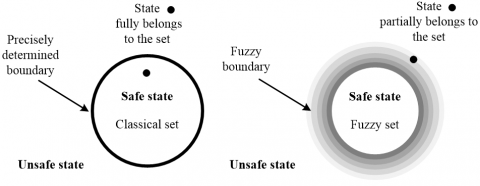Figure 1. The classic set and fuzzy set of safe state and unsafe state

The classic set separates the safe state from the unsafe state with clear and accurate boundaries. By contrast, the fuzzy set displays the smooth change form safe state to unsafe state, indicating that safety is a fuzzy problem. The fire hazard is an inherent problem of high-rise buildings. Thus, the fire safety of such building cannot be strictly classified as safe or unsafe. The true level of fire safety partly belongs to a state and partly belongs to another state. Here, the fuzzy situation is solved by the fuzzy logic system: the likelihood of a phenomenon was described by the membership function, and the fuzzy set theory was effectively integrated to the fire safety analysis, reducing the uncertainty of knowledge.

As shown in Figure 2, the fuzzy logic inference system involves three steps: fuzzification, fuzzy reasoning, and defuzzification.

## 2.png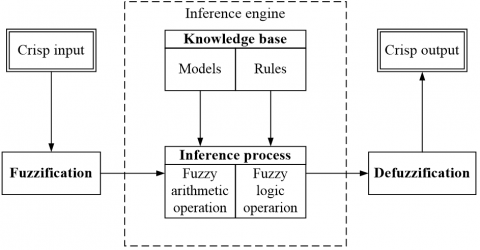Figure 2. Fuzzy logic inference system

(1) Fuzzification

To realize the fuzzy system, several fuzzy rules can be designed as:

Rule 1: If M is p1 & N is q1 & … then R is g1;

Rule 2: If M is p2 & N is q2 & … then R is g2.

where, Mi, Ni… are conditional variables; pi, qi, and gi… are fuzzy parameters obtained by membership function; R is the control output of fuzzy logic.

In the fuzzy rules, the condition variables are often configured by setting the membership of input point to 1 or configured based on the membership value. The Mamdani fuzzy inference method is an operation rule that combines fuzzy relation and fuzzy set. By this method, the membership values μp1(m), μq1(n), μp1(m) and μq1(n) in the range of D1 and D2 are matched with the corresponding fuzzy variables.

(2) Fuzzy reasoning

Let M = m and N = n. The control rules can be matched based on membership:

Rule Ⅰ: μ1 = μp1(m)^μq1(n);

Rule Ⅱ: μ2 = μp2(m)^μq2(n).

where, $\Lambda$ is a Mamdani inference method similar to the min function.

## 3.png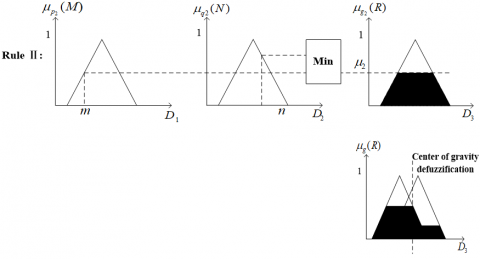Figure 3. The fuzzy reasoning process

The truth degrees of Rules I and II can be denoted as μ1 and μ2, respectively. Then, the fuzzy reasoning process can be described as Figure 3, where μg1(R) and μg2(R) are the defined fuzzy subsets g1(R) and g2(R) of m and n for measurement; D3 is the value range of output variable; g(R) is the fuzzy control output, i.e. the set of fuzzy subsets gi(R). The membership μgi(R) of g(R) is the sum of all membership values:

$\mu_{g}(R)=\mu_{c 1}(R) * \mu_{c 2}(R)$     (12)

where, * is a Mamdani inference method that takes the maximum value in the set.

(3) Defuzzification

Defuzzification aims to convert the fuzzy result (inference result) into a real value that can be used as a control input. The control output was obtained from μgi(R), because fuzzy result is not desired. The center of gravity method can be adopted for defuzzification:

$Z_{c}=\frac{\int_{z} Z \cdot \mu_{A}(Z) d z}{\int_{z} \mu_{d}(Z) d z}$     (13)

where, ∫z is the algebraic integral of the membership values of all elements in the subset output by fuzzy logic system on the continuous domain Z.

3.2 The RBFNN

Due to their excellence in generalization and learning, the ANNs have been extensively applied in various fields, ranging from including pattern recognition, flow prediction, industrial control, to evaluation. The most common ANN in safety assessment is the BPNN. However, the learning speed and convergence speed of the BPNN are far from ideal. As a result, the BPNN is easy to fall into the local minimum trap, which affects the accuracy of safety assessment. These defects of the BPNN could be overcome by the RBFNN, which is rarely applied to fire safety assessment of high-rise buildings.

## 4.png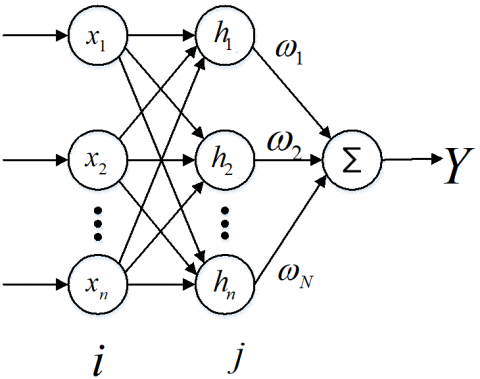Figure 4. The RBFNN

(1) Working principles

As shown in Figure 4, the RBFNN is a feedforward local approximation network with a single hidden layer. There are three layers in the RBFNN: input layer, hidden layer, and output layer [25-27]. The hidden layer nodes are usually activated by the Gaussian function, making the RBFNN radially symmetric about the center of the Gaussian function. Let $X \in R\left(X=\left[x_{1}, x_{2}, \ldots, x_{n}\right]^{T}\right)$ be the input vector. Then, the hidden layer output can be expressed as:

$h_{j}(x)=\exp \left(\frac{-\left\|X-r_{j}\right\|^{2}}{2 \sigma_{j}^{2}}\right), j=1,2, \ldots, N$     (14)

where, rj is the center of the Gaussian function in the hidden layer; $\sigma_{j}$ is the width of the Gaussian function.

When the input vector Xn is imported to the RBFNN, the network will output:

$Y\left(X_{n}\right)=\sum_{i=1}^{N} \omega_{i} h_{i}\left(X_{n}\right)$     (15)

where, $\omega_{i}$ is the connection weight between the hidden layer and output layer.

(2) Training algorithm for the RBFNN

The training algorithms of the ANNs are either unsupervised learning algorithm or supervised learning algorithm. Here, unsupervised learning is adopted to train the RBFNN. The center of the Gaussian function was determined by fuzzy C-means (FCM) clustering. In the FCM clustering, the data are classified based on the membership function $U=\left\{\mu_{i}^{p}\right\}_{c \times n},$ where $\mu_{i}^{p}$ is the membership of sample p relative to cluster center i. Then, the objective function κ can be processed as:

$\kappa\left(U, v_{1}, v_{2}, \cdots, v_{c}\right)=\sum_{i=1}^{N_{2}} \kappa_{i}=\sum_{i=1}^{N_{2}} \sum_{k=1}^{n}\left(\mu_{i}^{p}\right)^{L} d_{i p}^{2}$    (16)

where, $d_{i p}=\sqrt{\sum_{q=1}^{s}\left(x_{p q}-v_{i q}\right)^{2}}$ is the Euclidean distance between cluster center and fuzzy group; $L \in(1,+\infty), c \geq 2 ; u_{i p} \in(0,1)$.

Supervised learning, the key to the RBFNN, iteratively updates the network weights for a given network structure based on label information (desired output). This learning strategy ensures that the RBFNN operates according to the desired assessment method. In the error backpropagation training of the RBFNN, the network weights $\omega_{i}$ were trained by the gradient descent with a momentum factor. Let z(t) be the evaluation outputted by the RBFNN, and zm(t) be the evaluation made by experts. Then, the network weights can be adjusted by:

$\Delta \omega_{j}(t)=-\xi \frac{\partial E}{\partial w_{j}}=\xi\left(z(t)-z_{m}(t)\right) \alpha_{j}$      (17)

$\omega_{j}(t)=\omega_{j}(t-1)+\Delta \omega_{j}(t)+\gamma\left(\omega_{j}(t-1)-\omega_{j}(t-2)\right)$     (18)

where, $\xi \in(0,1)$ is the learning rate; $\gamma \in(0,1)$ is the momentum factor.

The RBFNN was trained into an intelligent fire safety evaluator by learning the sample data of the fire safety experts. The trained network mimics the assessment process of fire safety experts.

3.3 Fuzzy-RBFNN fire safety evaluation model

The fuzzy inference system was combined with the RBFNN into an adaptive fire safety assessment model for high-rise buildings (Figure 5). The proposed model was trained by numerous expert evaluation samples on fire safety of high-rise buildings. During the training, the center of Gaussian function and network weights were adjusted adaptively, making the model intelligent, accurate, and efficient.

For simplicity, the same function was adopted for the nodes on each layer. The structure and mechanism of the adaptive fuzzy-RBFNN are introduced below:

In the first layer (input layer), the nodes are directly connected to the input $\beta_{i}$ to pass the input vector $B=$ $\left[\beta_{1}, \beta_{2}, \ldots, \beta_{n}\right]$ to the next layer. There are $N_{1}=n$ nodes in this layer.

## 5.jpgFigure 5. The structure of adaptive fuzzy-RBFNN

In the second layer (membership function layer), each node represents a fuzzy set. If the membership function is defined as the Gaussian function, then the output function corresponding to each node constitutes the membership function of the fuzzy set:

$\beta_{i}^{j}=\exp \left[-\left(\beta_{i}-r_{i j}\right)^{2} / \sigma_{i j}^{2}\right]$    (19)

where, i=1,2,…,n; j=1,2,…,mi; rij and σij are the center and width of the Gaussian function, respectively. There are $N_{2}=\sum_{i=1}^{n} m_{i}$ nodes in this layer. The center of the membership function directly bears on the assessment result. Hence, the FCM clustering was performed on all input samples to determine the center rij of each Gaussian function. To assess the fire safety of high-rise buildings, the mean of the evaluated values of each level was taken as the initial value of rij.

In the third layer (fuzzy inference layer), each node stands for a fuzzy inference rule:

$\mu_{l}=\min \left(\beta_{1}^{i}, \beta_{2}^{i_{2}}, \ldots, \beta_{n}^{i_{n}}\right)$    (20)

where, $i_{1} \in\left\{1,2, \ldots, m_{1}\right\} \quad ; \quad i_{2} \in\left\{1,2, \ldots, m_{2}\right\} \quad, \ldots \quad i_{n} \in$ $\left\{1,2, \ldots, m_{n}\right\} ; l=1,2, \ldots, \prod_{i=1}^{n} m_{i} .$ The closer the input is to the center of the input variables, the greater its membership function. The inverse is also true. There are $N_{3}=m=\prod_{i=1}^{n} m_{i}$ nodes in this layer.

In the fourth layer (normalization layer), the results are normalized by:

$\phi_{i}=\frac{\beta_{i}}{\sum_{n=1}^{m} \beta_{n}}$      (21)

In the fifth layer (output layer), all signals are superimposed into the network output:

$y=\sum_{l=1}^{m} \omega_{k l} C_{l}$     (22)

where, Clis the value of consequent evaluation; ωkl is the matrix of weights in the adaptive fuzzy-RBFNN.

In the adaptive fuzzy-RBFNN, the connection weights between fuzzy inference layer and output layer, as well as the center and width of Gaussian function, were optimized through the training on expert evaluation samples based on the gradient descent with momentum factor. After the training, the proposed network fit better with the assessment rules of fire safety experts.

4. Simulation Verification

To verify the proposed network, a training set and a test set were developed based on the comments of fire safety experts on 22 high-rise buildings in a province of China. The expert evaluation samples of 14 high-rise buildings were used to train the proposed adaptive fuzzy-RBFNN, and those of 8 high-rise buildings were used to test the network. The center of membership function (Gaussian function) was determined by the FCM clustering algorithm based on the training set. The other parameters were configured as follows: the width of Gaussian function σ=3.2, the learning rate η=0.4, the momentum factor ξ=0.02, and the initial weight ωj=0.01. The trained adaptive fuzzy-RBFNN was adopted to assess the fire safety of the 8 high-rise buildings in the test set.

Figure 6 depicts the convergence of the training error of the adaptive fuzzy-RBFNN. Obviously, the assessment error of the proposed network meets the requirements after 14 iterations.

## 6.png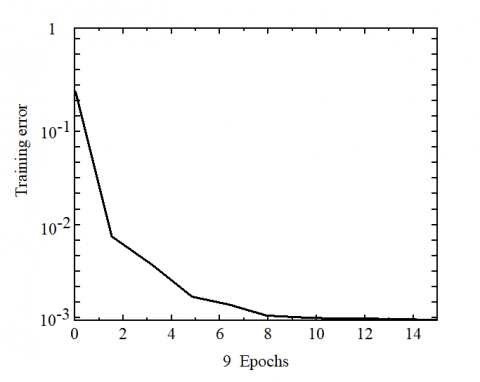Figure 6. The convergence of the training error

The evaluation results and relative errors of our network on the 8 test samples are listed in Table 5.

Table 5. Evaluation results and relative errors on the 8 test samples

 Samples Expert results Our results Relative errors 1 1.4 0.9 -35.7% 2 3.6 3.5 -2.8% 3 3.0 2.9 -3.3% 4 2.5 2.5 0 5 2.5 2.4 -4% 6 2.5 2.5 0 7 2.5 2.5 0 8 2.5 2.5 0

## 7.pngFigure 7. Comparison of evaluation results

## 8.png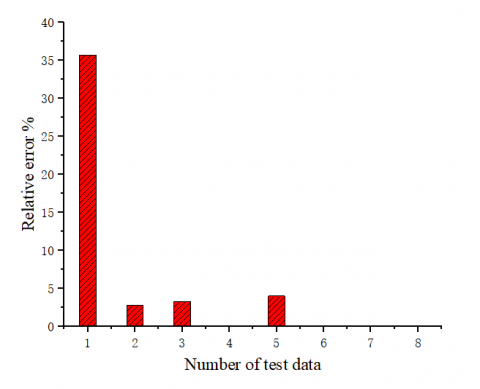Figure 8. Relative error of the BPNN

Meanwhile, the traditional BPNN was also applied to assess the 8 test samples. The results and relative errors of the BPNN are displayed in Figures 7 and 8, respectively. It can be seen that our network output similar results as expert evaluation, while the BPNN results exhibited the right trend but contained a high error. The excellence of our network is attributable to the following factors: through the learning and training of expert evaluation samples, the nonlinear evaluation process is converted into network data that can be understood by the evaluation model. The above results show that our network can generalize the logic of experts after training.

5. Conclusions

Targeting the fire safety assessment of high-rise buildings, this paper combines fuzzy theory and the RBFNN into a novel adaptive fuzzy-RBFNN model. The proposed method greatly improves the learning speed and simulation accuracy of the BPNN, and avoid the local minimum trap. Through the verification on actual high-rise buildings, our method was found to mimic the reasoning process of fire safety experts quickly and accurately. Compared with a single standard and the BPNN, the proposed adaptive fuzzy-RBFNN can evaluate the fire safety of high-rise buildings with high efficiency, accuracy, and reliability.

So far, the AI techniques like fuzzy theory-based RBFNN have not been widely applied to fire safety assessment of high-rise buildings, failing to provide sufficient experimental evidence. The future research will apply the proposed network to more high-rise buildings, and use the ample experimental data to enhance the stability and accuracy of our network. Furthermore, the fuzzy rules will be modified to make our network more applicable to fire safety assessment of engineering projects.

Acknowledgement

This paper was supported by 2019 Yiwu Industrial & Commercial College Preparatory Research Project of National (Project No: YY-GJ-201903).

References

 Li, J., Rismanchi, B., Ngo, T. (2019). Feasibility study to estimate the environmental benefits of utilising timber to construct high-rise buildings in Australia. Building and Environment, 147: 108-120. https://doi.org/10.1016/j.buildenv.2018.09.052

 Chen, X., Huang, J., Yang, H., Peng, J. (2019). Approaching low-energy high-rise building by integrating passive architectural design with photovoltaic application. Journal of Cleaner Production, 220: 313-330. https://doi.org/10.1016/j.jclepro.2019.02.137

 Yeung, J.F.Y., Chan, D.W.M. (2019). Developing a holistic fire risk assessment framework for building construction sites in Hong Kong, Journal of Construction Research, 65: 65881289.

 Shariff, G.N., Yong, J.C., Salleh, N. (2019). Risk assessment of building fire evacuation with stochastic obstructed emergency exit. 2019 4th International Conference and Workshops on Recent Advances and Innovations in Engineering (ICRAIE), pp. 1-5.

 Dong, P., Wang, S. (2019). Fire risk factor analysis of high-rise building based on Bayesian network and fuzzy fault tree. 2019 5th International Conference on Control, Automation and Robotics (ICCAR), pp. 599-603.

 Samrout, M., Yalaoui, F., Chatelet, E., Chebbo, N. (2005). New methods to minimize the preventive maintenance cost of series parallel systems using ant colony optimization. Reliab Eng Systaf, 89(3): 346-354. https://doi.org/10.1016/j.ress.2004.09.005

 Watts, J.M. (1995). Fire risk ranking. In: DiNenno PJ et al (eds) SFPE handbook of fire prevention engineering. National Fire Protection Association, Quincy, pp. 5:12-15:26

 Zheng, X., Hu, P., Gao, J. (2019) Application of BP Neural Network in Fire Risk Assessment of Comprehensive Shopping Mall. 2019 International Conference on Intelligent Computing, Automation and Systems (ICICAS), pp. 416-420.

 Chow, W.K., Wong, L.T., Kwan, E.C.Y. (1999). A proposed fire safety ranking system for old high-rise buildings in the Hong Kong special administrative region. Fire Mater, 23(1): 27-31. https://doi.org/10.1002/(SICI)1099-1018(199901/02)23:13.0.CO;2-R

 Han, Z., Weng, W., Zhao, Q., Ma, X., Liu, Q., Huang, Q. (2013). Investigation on an integrated evacuation route planning method based on real-time data acquisition for high-rise building fire. IEEE Transactions on Intelligent Transportation Systems, 14(2), 782-795. https://doi.org/10.1109/tits.2012.2237398

 Watts, J.M. (1995). Fuzzy fire safety. Fire Technol 31(3): 193-194. https://doi.org/10.1007/BF01039190

 Zadeh, L.A. (1965). Fuzzy set. Inf Contr, 8: 338-353. https://doi.org/10.1016/S0019-9958(65)90241-X

 Zadeh, L.A. (1973). Outline of a new approach to the analysis of complex systems and decision processes. IEEE Transactions on Systems, Man, and Cybernetics, (1): 28-44. https://doi.org/10.1109/TSMC.1973.5408575

 Paralikas, A.N., Lygeros, A.I. (2005). A multi-criteria and fuzzy logic based methodology for the relative ranking of the fire hazard of chemical substances and installations. Process Saf Environ Prot, 83(B2):122-134. https://doi.org/10.1205/psep.04236

 Tsai, C.H., Chen, C.W., Chiang, W.L., Lin, M.L. (2008). Application of geographic information system to the allocation of disaster shelters via fuzzy models. Eng Comput, 25(1/2): 86-100. https://doi.org/10.1108/02644400810841431

 Chen, C.W., Yeh, K., Chiang, W.L., Chen, C.Y., Wu, D.J. (2007). Modeling H-infinity control and stability analysis for structural systems using Takagi-Sugeno fuzzy model. Vib Contr, 13(1): 1519-1534. https://doi.org/10.1177/1077546307073690

 Hsiao, F.H., Chen, C.W., Liang, Y.W., Xu, S.D., Chiang, W.L. (2005). T-S fuzzy controller for nonlinear interconnected systems with multiple time delays. IEEE Trans Circuits Syst I Regul Papers, 52(1): 1883-1893. https://doi.org/10.1109/TCSI.2005.852492

 Liu, K.F.R. (2003). A possibilistic petri net model for diagnosing cracks in RC structures. Comput Aided Civ Infrastruct Eng, 18(6): 426-439. https://doi.org/10.1111/1467-8667.00328

 Lo, S.M., Hu, B.Q., Liu, M., Yuen, K.K. (2005). On the use of reliability interval method and grey relational model for fire safety ranking of existing buildings. Fire Technol, 41(4): 255-270. https://doi.org/10.1007/s10694-005-3732-9

 Lo, S.M. (1999). A fire safety assessment system for existing buildings. Fire Technol, 35(2): 131-152. https://doi.org/10.1023/A:101546382 1818

 Zhao, C.M., Lo, S.M., Lu, J.A., Fang, Z. (2004). A simulation approach for ranking of fire safety attributes of existing buildings. Fire Saf J, 39(7): 557-579. https://doi.org/10.1016/j.firesaf.2004.06.001

 Xia, D., Kang, Q., Jia, D., Cheng, X., Liu, J. (2009). Application of fuzzy centralization statistical theory to fire risk assessment for high-rise building. In 2009 Sixth International Conference on Fuzzy Systems and Knowledge Discovery, 3: 59-63. https://doi.org/10.1109/FSKD.2009.298

 Geramian, A., Shahin, A., Minaei, B., Antony, J. (2020). Enhanced FMEA: An integrative approach of fuzzy logic-based FMEA and collective process capability analysis. Journal of the Operational Research Society, 71(5): 800-812. https://doi.org/10.1080/01605682.2019.1606986

 Nilashi, M., Yadegaridehkordi, E., Ibrahim, O., Samad, S., Ahani, A., Sanzogni, L. (2019). Analysis of Travellers’ online reviews in social networking sites using fuzzy logic approach. International Journal of Fuzzy Systems, 21(5): 1367-1378. https://doi.org/10.1007/s40815-019-00630-0

 He, Q.F., Shahabi, H., Shirzadi, A., Li, S., Chen, W., Wang, N., Chai, H.C., Bian, H.Y., Ma, J.Q., Chen, Y.T., Wang, X.J., Chapi, K., Ahmad, B.B. (2019). Landslide spatial modelling using novel bivariate statistical based Naïve Bayes, RBF Classifier, and RBF Network machine learning algorithms. Science of the Total Environment, 663: 1-15. https://doi.org/10.1016/j.scitotenv.2019.01.329

 Flyer, N., Fornberg, B., Bayona, V., Barnett, G.A. (2016). On the role of polynomials in RBF-FD approximations: I. Interpolation and accuracy. Journal of Computational Physics, 321: 21-38. https://doi.org/10.1016/j.jcp.2016.05.026

 Hoori, A.O., Motai, Y. (2017). Multicolumn RBF network. IEEE Transactions on Neural Networks and Learning Systems, 29(4): 766-778. https://doi.org/10.1109/TNNLS.2017.2650865